Courses

# Laws of Thermodynamics and Enthalpy Class 11 Notes | EduRev

## Class 11 : Laws of Thermodynamics and Enthalpy Class 11 Notes | EduRev

The document Laws of Thermodynamics and Enthalpy Class 11 Notes | EduRev is a part of the Class 11 Course Chemistry for JEE.
All you need of Class 11 at this link: Class 11

LAWS OF THERMODYNAMICS
Zeroth Law
Based on thermal equilibrium if A & B, & B & C are in thermal equilibrium then A & C must be in thermal equilibrium.

First Law of Thermodynamics
First law of thermodynamics is based on energy conservation

E= E1 + q + w (E1 is the Ei)
E- E= q + w (E1 q w is Ef)
(Ei = Ef)

 ΔE = Q+w

For an isolated system, q = 0, w = 0
ΔU = 0
or U = constant
For cyclic process.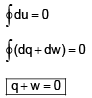Work done = - Fext.dx
= - P. A dx

 dW = -PextdV

for expansion → dW = - ve
compression → dW = +ve
dq = CdT
⇒ dqv = CvdT
Cv = dqv/dT = qv/ΔT
For an isochoric process dv = 0
dU = qV
⇒ dU =  CdTa
dU = n CVdT
ΔU = nCv(T2 - T1)
If CV is a function of temperature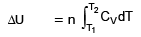We know that
U = f(T, V, P)
consider U = f(T, V)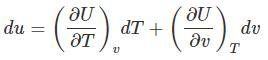For isochoric process dv = 0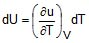for 1 mole of gas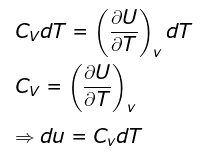For an ideal gas U = f(T) only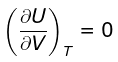du = CVdT
ΔU = nCVΔT = nCV(T2 - T1)

 ΔU = nCVΔT = nCV(T2 - T1)

ENTHALPY
H = U PV
dH = dU d(PV)
∫dH =  ∫dU + P∫dV
at constant pressure
⇒ ΔH = ΔU + PΔV

From, Ist law of thermodynamics at constant pressure.
dU = dqp + dW
dU = dqp - PdV
dqp = dU + PdV
dH = dU + PdV
ΔH = ΔU + PΔV  ....(1)
at constant volume
ΔH = Δ U +  VΔP ..........(2)

When P & V both changes
ΔH = Δ U +  (P2V2 - P1V1) ..........(3)
ΔH = Δ U + d(PV)
ΔH = Δ U + d(nRT)
ΔH = Δ U + RTDng

For an ideal gas expansion or compression

 ΔH = ΔU + nRΔT

ΔH = nCVΔT +  nRΔT
= nΔT[CV  + R]

 ΔH = nΔT Cp

We know that, H = f(T, P)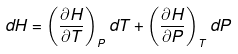At constant pressure
dP = 0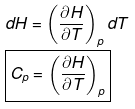Calculation of Δng for any chemical reaction: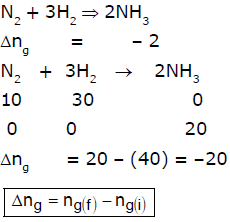If reaction is 50% completed.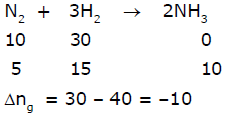Offer running on EduRev: Apply code STAYHOME200 to get INR 200 off on our premium plan EduRev Infinity!

## Chemistry for JEE

223 videos|452 docs|334 tests

,

,

,

,

,

,

,

,

,

,

,

,

,

,

,

,

,

,

,

,

,

;